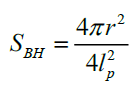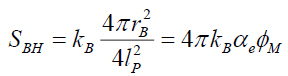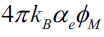All submissions of the EM system will be redirected to Online Manuscript Submission System. Authors are requested to submit articles directly to Online Manuscript Submission System of respective journal.

# Original Article

, Volume: 11( 5) DOI: 10.37532/2320-6756.2023.11 (5).345

## Redefining Black Hole Entropy Due to Proportionality to the Fine Structure Constant

*Correspondence:
Keith Huckelby Independent Researcher, USA E-mail: keithhuck7000@gmail.com

Received date: 12-May-2023, Manuscript No. tspa-23-98430; Editor assigned: 14-May-2023, Pre-QC No. tspa-23-98430 (PQ); Reviewed: 22- May-2023, QC No. tspa-23-98430 (Q); Revised: 25-May-2023, Manuscript No. tspa-23-98430 (R); Published: 27-May-2023, DOI. 10.37532/2320-6756.2023.11 (5).345

Citation: Huckelby K. Redefining Black Hole Entropy Due to Proportionality to the Fine Structure Constant. J. Phys. Astron.2023;11(5):345.

### Abstract

In this article we propose a new definition of the entropy of black holes. For this we base ourselves on a reformulation of the HawkingBekenstein formula in such a way that, on the one hand, by introducing a new variable that represents the number of microstates and On the other hand, taking into account the mass of the black hole, we can conclude a new equation for the entropy of black holes.

### Keywords

Black hole; Entropy; Microstates; Black hole mass

### Introduction

In this paper I will present an equation which will show how the black hole entropy is proportional to the fine structure constant. Afterwards I will explain how this equation relates to black hole entropy and how we can define it in relation to the original Hawking-Bekenstein black hole entropy formula. We can see below the original formula,The equation above tells us the amount of entropy of a black hole. Originally Bekenstein derived this formula and concluded a black hole entropy is proportional to the black hole’s event horizon surface area.

The Fine Structure ConstantAbove we have an equation which gives us the value of the fine structure constant. We represent the fine structure constant as. The variablecan also be given by the second expression.This expression essentially implies that the fine structure constant is a probability which shows the chance of an electromagnetic interaction between a photon and an electron within an atomic electrostatic interaction. The probability is one out of 137 atomic electrostatic interactions emitting or absorbing a photon.

The New Micro-State Variable for Black Hole EntropyThis is the micro-state variable we will use in our new black hole entropy equation. The variable tells us how many atomic electrostatic interactions are needed within a certain surface area to result in the gravitational field of the mass “m”. Keep in mind the surface area is derived using the Schwarzschild radius of the mass "m". Whenever the mass “m” equals the Planck mass,equals which is the maximum of the fine structure constant .

Multiplying the fine structure constant and the micro-state variable,The product of both the fine structure constant αe and the micro-state variablegive us the squared number of Planck masses "mP" within an overall mass "m". Within each Planck mass there are 137 atomic electro-static interactions and each 137 electrostatic interaction there are at least one of the interactions has an electro-magnetic absorption or emission of a photo.

Presenting a New Form of Black Hole EntropyThis is the black hole entropy equation in its original form reduced to. The original Hawking-Bekenstein equation makes the black hole entropy proportional to the surface areawhere "rB" is the Schwarzschild radius of the mass “m”."rB"equals 2Gm divided by c2.The new form makesthe micro-state variable proportional to the black hole entropy SBH.

Keep in mindis the fine structure constant andequals.

This new form of black hole entropy needs to be interpreted because of how I have defined above the variables of the fine structure constant and the micro-state .

I conclude black hole entropy in this new form can be interpreted as a measure of the different states in which a certain number of atomic electro-static interactions can be arranged in a Schwarzschild surface area to result in the gravitational field of mass “m”.

List of Physical Parameters

 SB Black hole entropy kB Boltzmann constant, 1.3807×10-23JT-1 c Speed of light , 299792458 ms-1 G Gravitational constant, 6.674×10-11m3kg-1s-2 m Mass of black hole rb Schwarzschild radius of mass “m” Ip Planck length, 1.6162×10-35mMicro-state variable of black hole entropy mP Planck mass, 2.176×10-8 kg

### REFERENCES

1. Penrose Roger. Vintage books. 2005
2. Alberto Coe. Micro Black Hole Entropy and Fine Structure Constant: A Numerological Approach. 2021
Welcome Message

Select your language of interest to view the total content in your interested language

###### Citations : 291

Journal of Physics & Astronomy received 291 citations as per Google Scholar report

#### Indexed In

• Open J Gate
• China National Knowledge Infrastructure (CNKI)
• Cosmos IF
• Directory of Research Journal Indexing (DRJI)
• MIAR
• Secret Search Engine Labs
• Euro Pub
• clarivate-web-of-science-logo-vector
• ICMJE

View More

Berlin, Germany

### World Congress on Quantum Physics

Berlin, Germany